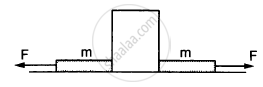# The Figure Shows a Heavy Block Kept on a Frictionless Surface and Being Pulled by Two Ropes of Equal Mass M. At T = 0, the Force on the Left Rope is - Physics

MCQ

The figure shows a heavy block kept on a frictionless surface and being pulled by two ropes of equal mass m. At t = 0, the force on the left rope is withdrawn but the force on the right end continues to act. Let F1 and F2 be the magnitudes of the forces exerted by right rope and the left rope on the block, respectively.#### Options

• F1 = F2 = F for t < 0

• F1 = F2 = F + mg for t < 0

• F1 = F, F2 = F for t > 0

• F1 < F, F2 = F for t > 0

#### Solution

F1 = F2 = F, for t < 0
At t < 0, the block is in equilibrium in the horizontal direction.
So, F1 = F2 = F
At t > 0, F2 = 0 and F1 = F.

Concept: Newton's Third Law of Motion
Is there an error in this question or solution?

#### APPEARS IN

HC Verma Class 11, Class 12 Concepts of Physics Vol. 1
Chapter 5 Newton's Laws of Motion
MCQ | Q 5 | Page 78